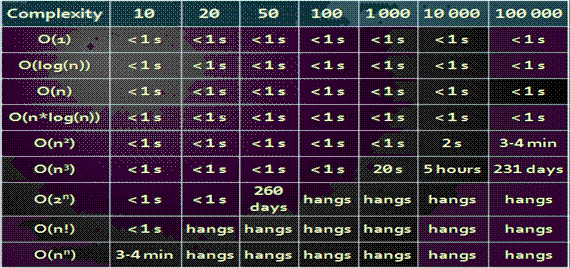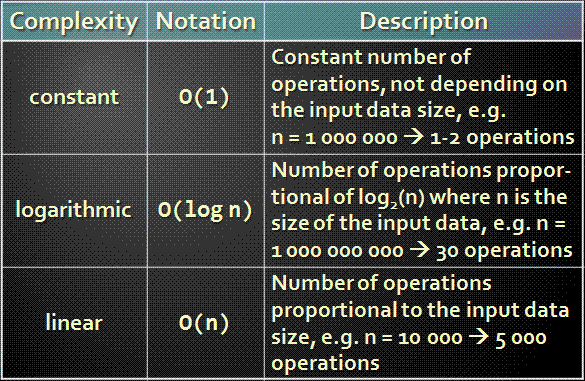# Complexity Data Structure Assignment Help

## Complexity Data Structure

The complexity of an algorithm is the function which gives the running time and/or space in terms of the input size.

Time Complexity

1. Worst-Case
• An upper bound on the running time for any input of given size
2. Average-Case
• Assume all inputs of a given size are equally likely
3. Best-Case
• The lower bound on the running time

Algorithm Complexity is rough estimation of the number of steps performed by given computation depending on the size of the input data

• Measured through asymptotic notation
• O(g) where g is a function of the input data size
• Examples:
• Linear Complexity O(n) : All elements are processed once (or constant number of times)
• Quadratic Complexity O(n2) : Each of the elements is processed n times### Typical Complexities### Time And Memory Complexity

• Complexity can be expressed as formula on multiple variables, e.g.
• Algorithm filling a matrix of size n * m with natural numbers 1, 2, … will run in O(n*m)
• DFS traversal of graph with n vertices and m edges will run in O(n + m)
• Memory consumption should also be considered, for example:
• Running time O(n), memory requirement O(n2)
• n = 50 000 Ĺ• OutOfMemoryException

### Find the best Trees Complexity Data Structure Assignment Help Services with us

Try our determination care now, solution of your problem is righteous a depression departed. Knock any quantify at our 24x7 live supports for any ask. To know about how to proceed, just visit how it Works page at Assignmenthelp.net.